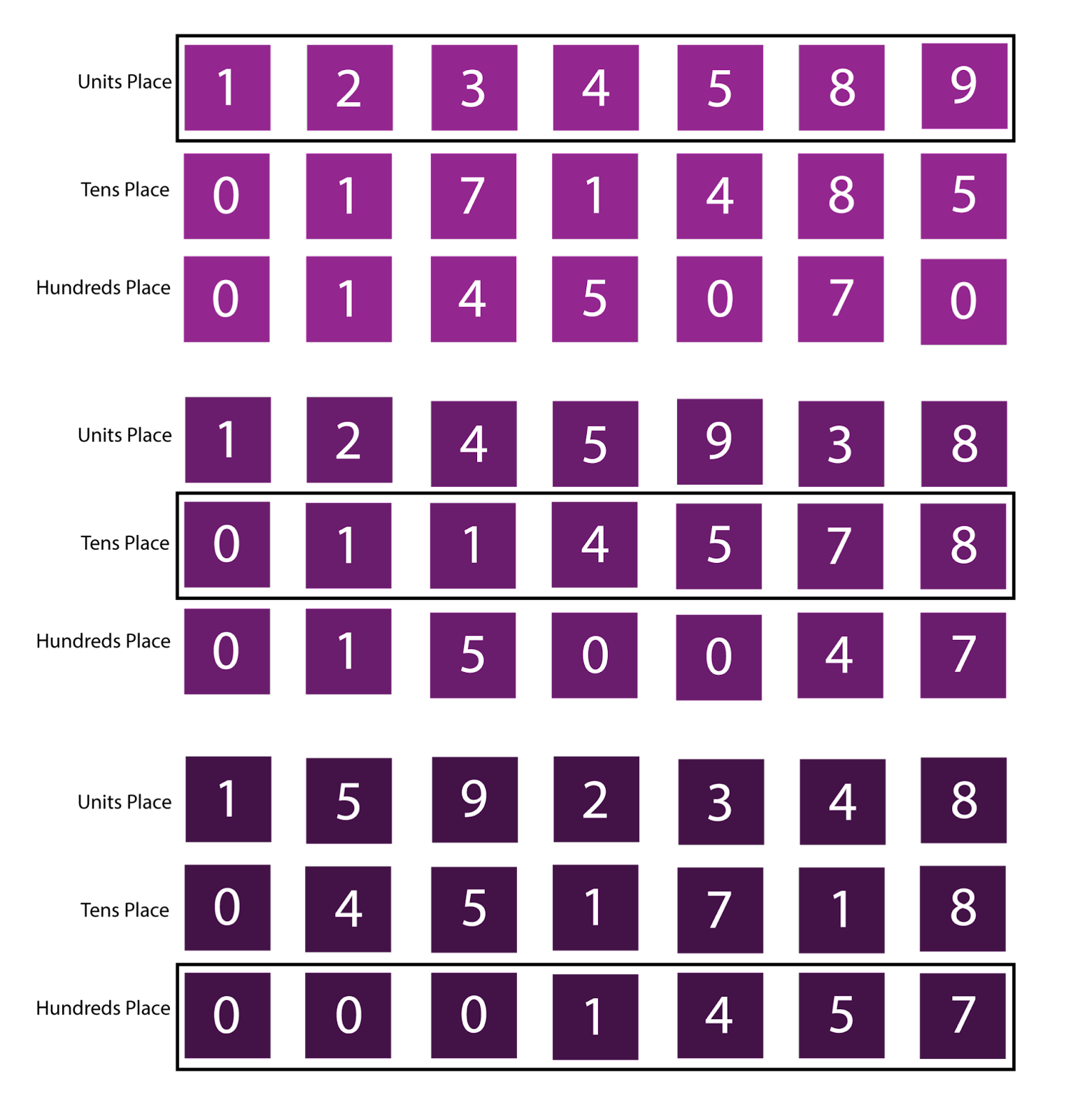## Introduction

Understanding Radix Sort will require a good understanding of counting sort, which you can study here, and then this article will proceed to explain the working; and with an example, the algorithm and code for Radix Sort. Further, there will be a discussion about time complexity followed by some questions related to the topic.

## What is Radix sort used for?

Radix sort is known as an integer sorting algorithm that sorts elements by individually grouping digits based on their face value. Being a non-comparative sorting algorithm, it distributes its elements to avoid comparison.

Radix sort comes in handy when the range of elements is N ^ 2, and the implementation of counting sort cannot be implemented because it is only efficient for elements in the range 1 to ‘K’, where ‘K’ is the maximum element.

## Radix sort from counting sort

Radix sort is of two types-LSD (least significant digit), where it starts from the end of the string (least significant value), or MSD (most significant digit), where the sorting starts from the beginning of the string(most significant value).

In the given example, we will be using LSD-the elements will first be sorted based on their unit place using counting sort, then their tens place and so on till the most significant place.

Let’s consider an array of elements 112, 473, 514, 59, 1, 45, 788 and use counting sort to sort the elements beginning from the units place:## Algorithm

• The maximum element of the initial array is stored in variable ‘K’.
• ‘Count’ array is created with all elements initially being 0, and then the count is incremented every time the number at the index occurs in the array.
• Next, we calculate the cumulative count and the elements are placed in sorted order after counting sort.
• A function is made to retrieve the largest element in the array and then finally a function is made to implement radix sort after counting sort.
• In this function, the maximum element is stored and elements are sorted bases on place value.
• The function is then called and the sorted array is printed.

## Time Complexity

The time complexity of radix sort isT(N) = O(D * (N + B) ), ‘D’ being the number of digits in a number, ‘N’ being the number of elements, and ‘B’ being the base or bucket size. Usually, base 10 is used for decimal representation.

Radix sort is mostly faster than O(N * (log N)) and hence better.

## Space Complexity

The space complexity for Radix sort is  N + K, ‘K’ denoting the number of digits in the maximum element in the array.

Radix sort uses Counting sort for each digit of numbers in the array. Counting sort has space complexity of O(N + K), as Counting Sort using an auxiliary space to sort every 10th place digit

1. Can insertion sort be used as an intermediate sort for Radix sort?

Radix Sort can use any of the following to sort individual digits: Bubble sort, counting sort, insertion sort or bucket sort. This is because the runtime is O(N * K), ‘K’ being the maximum number and ‘N’ being the length of the array.

2. Is Quick sort better than Radix Sort?

Quick sort has an average complexity of N * log(N) and usually the lowest ‘K’ among all techniques. Radix sort however has a large value for ‘K’ and therefore quick sort is generally better and faster than Radix sort.

3. Can Radix Sort be used on strings?

Radix sort treats integers as strings of digits. So, despite being developed for large integers, it is actually a string sorting technique. Radix sort can either be LSD (least significant digit) or MSD (most significant digit).

The Radix Sort algorithm is dependent on letters or digits, therefore making it less flexible than other techniques. Moreover, the constraint for this technique is larger in comparison to the other techniques, and thus Radix sort takes up more space than in-place sorting techniques like Quicksort.

## Key takeaways

In this article, we learned how the radix sort algorithm works and how it is implemented, along with an example.

The Radix sort algorithm is very efficient for cases where input values are lesser than or equal to the number of values to be sorted. You can go to CodeStudio and try solving problems. Share this blog with your friends if you found it helpful!

Until then, All the best for your future endeavours, and Keep Coding.